ML Aggarwal Class 7 Solutions Chapter 7 Percentage and Its Applications Ex 7.3 for ICSE Understanding Mathematics acts as the best resource during your learning and helps you score well in your exams.

## ML Aggarwal Class 7 Solutions for ICSE Maths Chapter 7 Percentage and Its Applications Ex 7.3

Questions 1.
Rohan bought a calculator for ₹ 760 and sold it for ₹ 874. Find his profit and profit percentage.
Solution: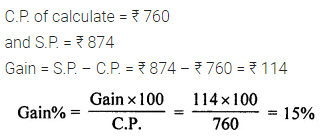Question 2.
Kirti bought a saree for ₹ 2500 and sold it for ₹ 2300. Find her loss and loss percent.
Solution: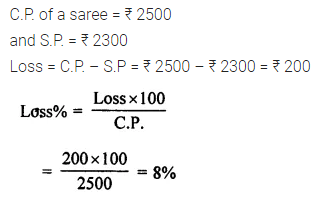Question 3.
Tell what is profit or loss in the following transactions. Also find profit percent or loss percent in each case:
(i) Gardening shears bought for ₹ 250 and sold for ₹ 325.
(ii) A shirt bought for ₹ 250 and sold at ₹ 150.
Solution: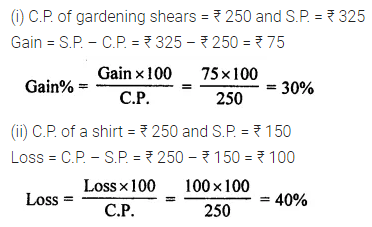Question 4.
Rajinder bought one almirah for ₹ 4800 and the other for ₹ 3640. He sold the first almirah at a gain of 13$$\frac { 1 }{ 3 }$$ % and the other at a loss of 15%. How much did he gain or lose in the whole deal?
Solution: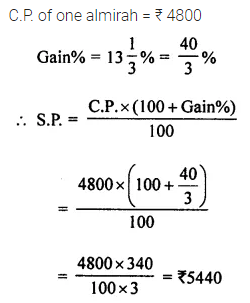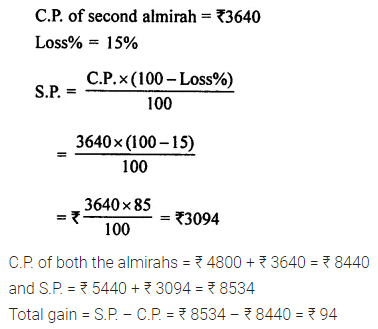Question 5.
In a furniture shop, 24 tables were bought at the rate of ₹ 450 per table. The shopkeeper sold 16 of them at the rate of ₹ 600 per table and the remaining at the rate of ₹ 400 per table. Find his gain or loss percent.
Solution:Question 6.
By selling a fan for ₹ 810, a dealer makes a profit of ₹ 60. What is the cost price of the fan? What is his profit percent?
Solution:Question 7.
By selling a steel almirah for ₹ 3906, a manufacturer suffers a loss of ₹ 294. Find the cost price of the almirah and his loss percentage.
Solution: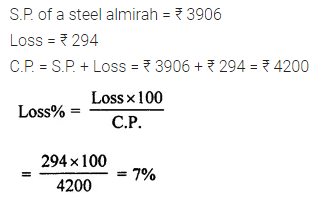Question 8.
The cost price of a flower vase is ₹ 120. If the shopkeeper sells it at a loss of 10%, find the price at which it was sold.
Solution:Question 9.
I buy a T.V. for ₹ 10000 and sell it at a profit of 20%. How much money do I get for it?
Solution:Question 10.
A shopkeeper sells an article at ₹ 300, thus earning a profit of 20%. Find the cost price of the article.
Solution: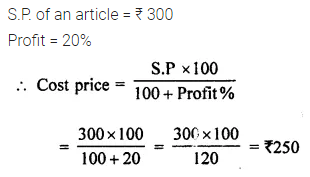Question 11.
A shopkeeper sells i n article at ₹ 320, thus suffering a loss of 20%. Find the cost price of the article.
Solution: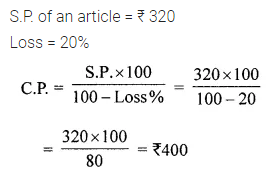Question 12.
By selling a chair for ₹ 522, a shopkeeper makes a profit of 16%. What is its cost price?
Solution: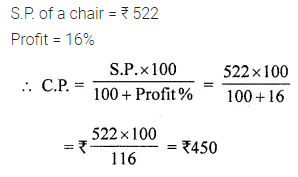Question 13.
A trader sold some damaged garments for ₹ 7360 at a loss of 8%. Find the cost price of the garments.
Solution:Question 14.
By selling a table for ₹ 3168, Rashid loses 12%. Find its cost price. What percent would he gain or lose by selling the table for ₹ 3870?
Solution: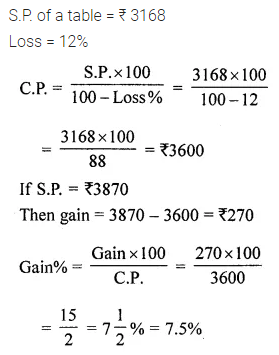Question 15.
By selling an article for ₹ 4550, Tony incurs a loss of 9%. What percent would he gain or lose by selling it for ₹ 4825?
Solution: